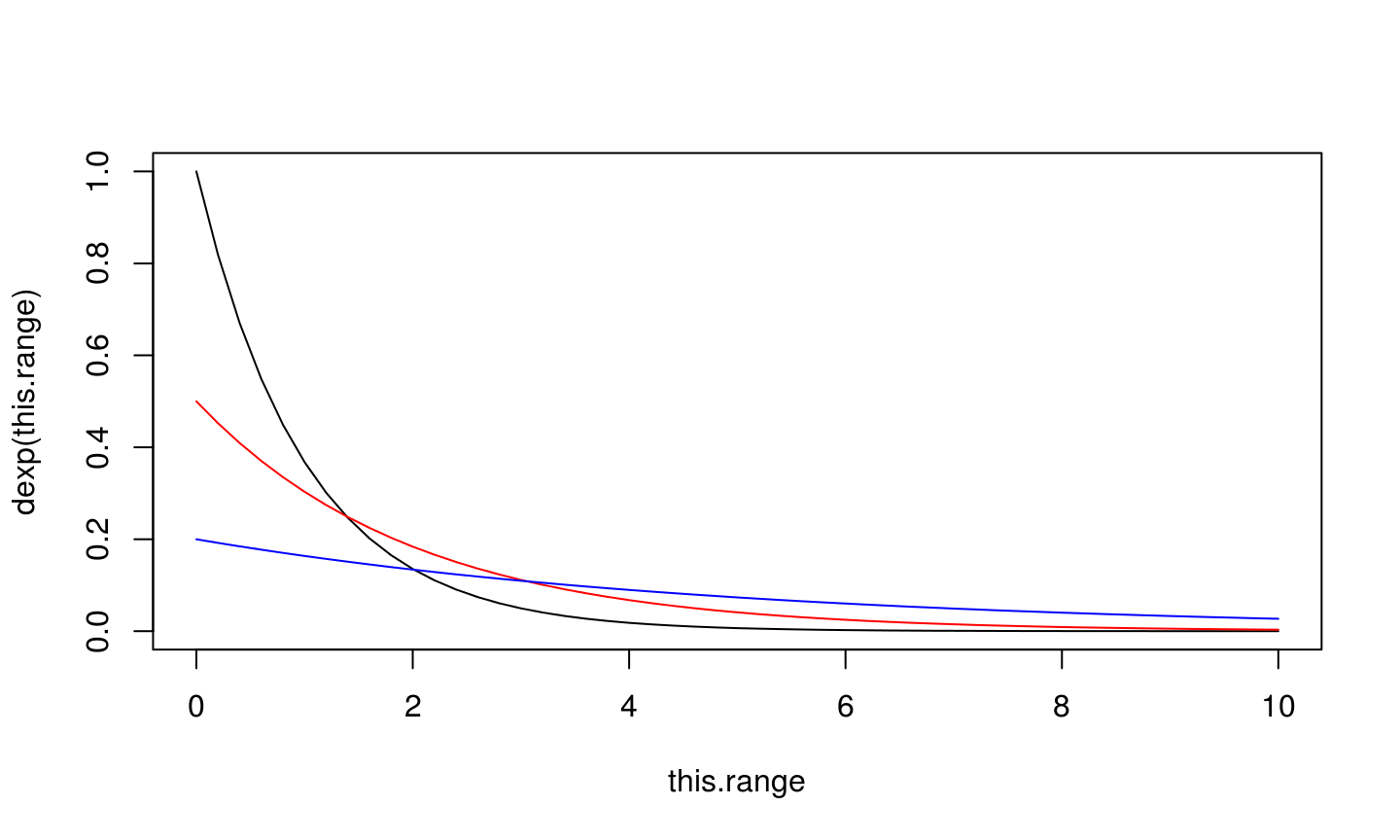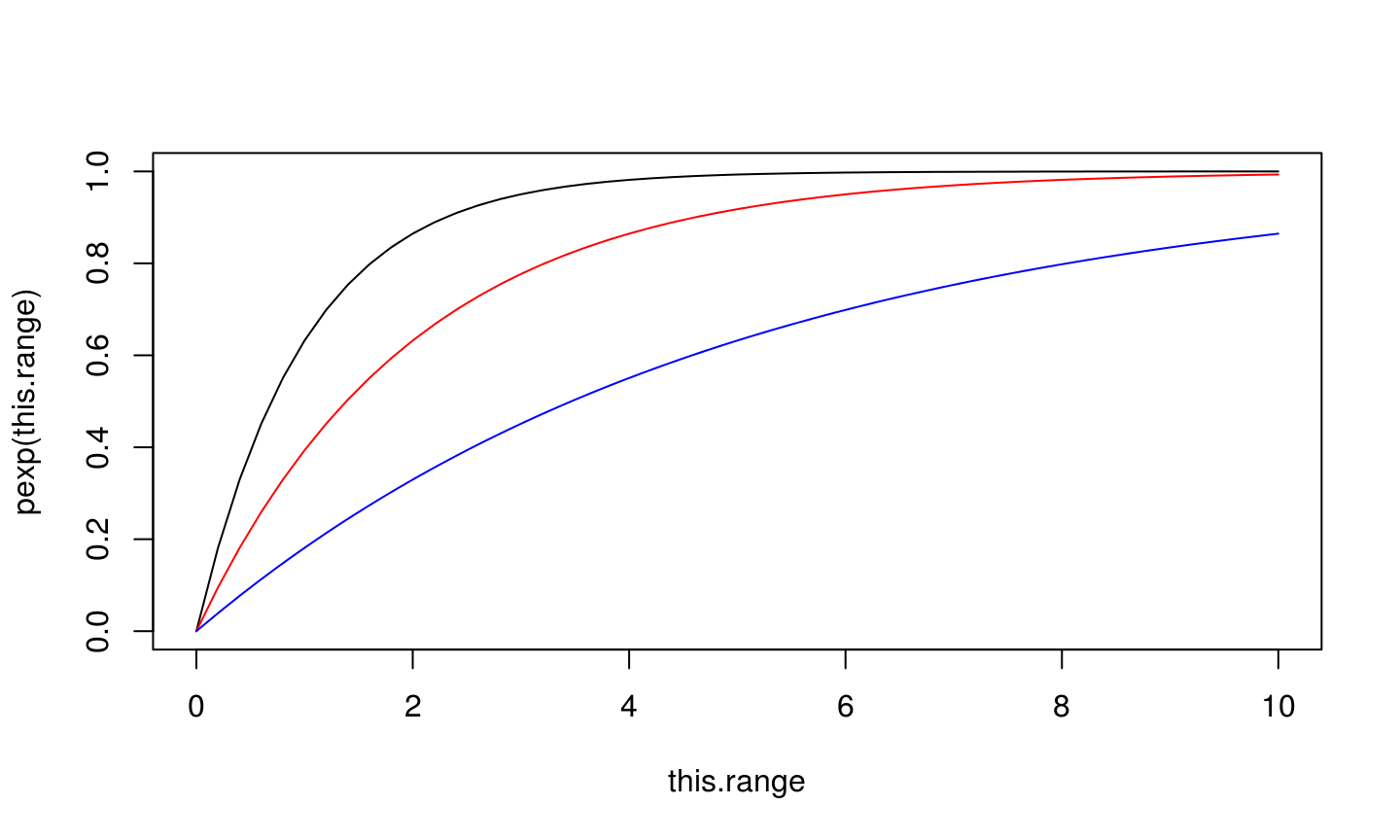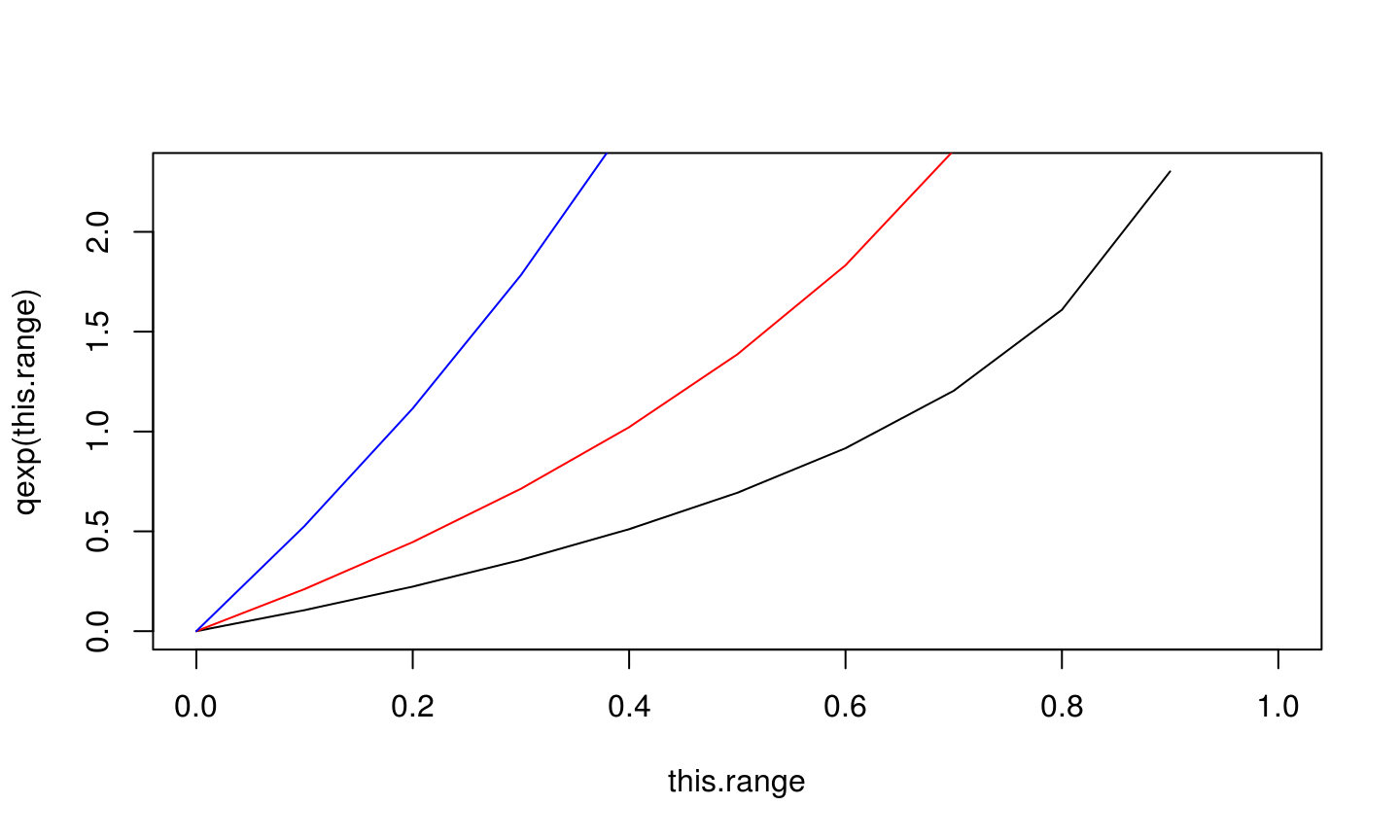29 September 2014

## In Previous Episodes

• We made reference to random number generation without going under the hood.

## Today

• How does R get "random" numbers, anyway?
• It doesn't, really – it uses a trick that should be indistinguishable from the real McCoy

## How Do We Get "Real" Randomness?

Introduce real-world noise:

• Thermal detection – trailing decimal points on a thermometer
• From Space! – cosmic ray/radioactive decay arrival timings, or any homogeneous Poisson process
• From Earth! – http://www.random.org/

## These Cost Money and I'm Cheap

Pseudorandom generators produce a deterministic sequence that is indistiguishable from a true random sequence if you don't know how it started.

## Example: runif, where we know where it started

runif(1:10)
##   0.20972 0.65726 0.70948 0.70757 0.56600 0.54488 0.70300 0.09676
##   0.63422 0.21623
set.seed(10)
runif(1:10)
##   0.50748 0.30677 0.42691 0.69310 0.08514 0.22544 0.27453 0.27231
##   0.61583 0.42967
set.seed(10)
runif(1:10)
##   0.50748 0.30677 0.42691 0.69310 0.08514 0.22544 0.27453 0.27231
##   0.61583 0.42967

## Basic version: Linear Congruential Generator

seed <- 10
new.random <- function (a=5, c=12, m=16) {
out <- (a*seed + c) %% m
seed <<- out
return(out)
}
out.length <- 20
variates <- rep (NA, out.length)
for (kk in 1:out.length) variates[kk] <- new.random()
variates
##   14  2  6 10 14  2  6 10 14  2  6 10 14  2  6 10 14  2  6 10

## Try again

Period 8:

variates <- rep (NA, out.length)
for (kk in 1:out.length) variates[kk] <- new.random(a=131, c=7, m=16)
variates
##    5  6  9  2 13 14  1 10  5  6  9  2 13 14  1 10  5  6  9  2

## Try again, again

Period 16:

variates <- rep (NA, out.length)
for (kk in 1:out.length) variates[kk] <- new.random(a=129, c=7, m=16)
variates
##    9  0  7 14  5 12  3 10  1  8 15  6 13  4 11  2  9  0  7 14

## Try again, at last

Numerical Recipes uses

variates <- rep (NA, out.length)
for (kk in 1:out.length) variates[kk] <- new.random(a=1664545, c=1013904223, m=2^32)
variates
##   1.037e+09 2.091e+09 4.106e+09 7.684e+08 3.836e+09 1.329e+09 2.125e+09
##   2.669e+09 3.582e+09 2.079e+09 2.067e+09 2.197e+09 3.749e+09 2.914e+09
##  7.588e+08 4.029e+09 2.837e+09 1.458e+09 2.399e+09 2.767e+09

## How To Distinguish Non-Randomness

• We've covered period: if it's missing some values, it's distinguishable
• Uniformity of distribution in the limit
• Autocorrelation
• Dimensional distribution – not a problem for 1-D distributions, but can be for 2+-D

## How does R get everything we need?

A few distributions of interest:

• Uniform(0,1)
• Bernoulli(p)
• Binomial(n,p)
• Gaussian(0,1)
• Exponential(1)
• Gamma(a)

## In R: everything we need

Suppose we were working with the Exponential distribution.

• rexp() generates variates from the distribution.
• dexp() gives the probability density function.
• pexp() gives the cumulative distribution function.
• qexp() gives the quantiles.

## dexp()

dexp(0:5)
##  1.000000 0.367879 0.135335 0.049787 0.018316 0.006738
this.range <- 0:50/5
plot (this.range, dexp(this.range), ty="l")
lines (this.range, dexp(this.range, rate=0.5), col="red")
lines (this.range, dexp(this.range, rate=0.2), col="blue")## pexp()

pexp(0:5)
##  0.0000 0.6321 0.8647 0.9502 0.9817 0.9933
this.range <- 0:50/5
plot (this.range, pexp(this.range), ty="l")
lines (this.range, pexp(this.range, rate=0.5), col="red")
lines (this.range, pexp(this.range, rate=0.2), col="blue")## qexp()

qexp(0:5)
## Warning: NaNs produced
##    0 Inf NaN NaN NaN NaN
this.range <- seq(0,1,by=0.1)
qexp(this.range)
##   0.0000 0.1054 0.2231 0.3567 0.5108 0.6931 0.9163 1.2040 1.6094 2.3026
##     Inf
plot (this.range, qexp(this.range), ty="l")
lines (this.range, qexp(this.range, rate=0.5), col="red")
lines (this.range, qexp(this.range, rate=0.2), col="blue")